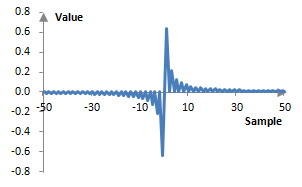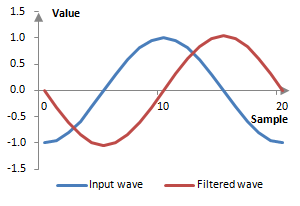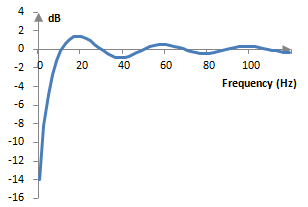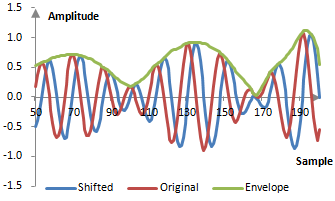# Hilbert transform

Much of digital signal processing requires working with negative frequencies. Negative frequencies in practice do not mean anything and introducing such frequencies in digital signal processing analysis may be troublesome. It is easy to convert a signal that contains negative frequencies into one that does not.

A converter that removes negative frequencies from an analytical signal is called a Hilbert transform.

Consider the following complex signal x(t) which contains both the positive and negative frequencies ω and -ω.

$$x(t) =e^{j\,\omega\,t}+e^{-j\,\omega\,t}$$

A good Hilbert transform H(x(t)) is something that would shift the signal by -π / 2 for positive frequencies and by π / 2 for negative frequencies.

$$H(x(t)) =e^{j\,\omega\,t-\frac{\pi}{2}}+e^{-j\,\omega\,t+\frac{\pi}{2}}=-j\,e^{j\,\omega\,t}+j\,e^{-j\,\omega\,t}$$

Then

$$x(t)+j\,H(x(t)) =e^{j\,\omega\,t}+e^{-j\,\omega\,t}-j^2\,e^{j\,\omega\,t}+j^2\,e^{-j\,\omega\,t}=2\,e^{j\,\omega\,t}$$

Thus, a Hilbert transform, multiplied by j and added to the original signal is of analytical interest, as it can be used to convert a signal containing positive and negative frequencies into a signal containing only positive frequencies. This Hilbert transform is also of practical interest, as it shifts all signals of positive frequencies by -π / 2, or a quarter of their cycle.

Finite impulse response (FIR) and infinite impulse response (IIR) filters that work as or at least approximate discrete Hilbert transforms exist. One way to design such a filter is to use its desired frequency response.

$$H(\omega)=\begin{cases} e^{j \frac{\pi}{2}}, \omega \lt 0 \\ 0, \omega=0 \\ e^{-j \frac{\pi}{2}}, \omega \gt 0 \end{cases}$$

or, more appropriately, the discrete version (for N odd)

$$H(k)=\begin{cases} j, k=1,2,...,\frac{N-1}{2} \\ 0, k=0 \\ -j, k=\frac{N+1}{2}, ..., N-1 \end{cases}$$

and to apply the inverse discrete Fourier transform. We get the Hilbert transform

$$a(k)=\frac{1}{N} (\cot(\frac{\pi\,k}{2})-\frac{\cos(\pi\,k)}{\sin(\frac{\pi\,k}{N})})$$

When N is even, we can similarly obtain

$$a(k)=\frac{2}{N} \sin^2(\frac{\pi\,k}{2})\,\cot(\frac{\pi\,k}{2})$$

In both cases, when N is odd and when N is even, a(k) = 0 for k = 0, 2, 4, ….

For very short transforms (e.g., N = 10), the Hilbert transform can be approximated by

$$a(k)=\begin{cases} 0, k \,even \\ \frac{2}{\pi\,k}, k\, odd \end{cases}$$

A Hilbert transform FIR filter of 101 points is shown below.The impact of this filter on a simple wave at 100 Hz sampled at 2000 Hz is shown on the figure below. This filter shifts the wave by a quarter of its cycle.Since the filter is finite and thus imperfect, however, it does not preserve the magnitude of all frequencies. The magnitude response of the example filter for the beginning of the frequency spectrum is as follows.This filter would exhibit similar loss of amplitude close to half of the sampling frequency. It is a band pass filter. This amplitude loss on both ends would be smaller for filters of larger length, and it would disappear faster for the upper end of the frequency spectrum and slower at the lower end. The magnitude responses of the Hilbert transform filters also show the characteristic Gibbs phenomenon ripples, which can be modified through windowing with the same windows applied to other FIR filters.

## Using the Hilbert transform

The fact that the Hilbert transform filter shifts all frequencies of the signal by -π / 2 makes it ideal for use in envelope detection. The amplitude envelope of a frequency can be computed with the square root of the sum of the squared value of the frequency at the specific sample and the squared value of the Hilbert transform of the frequency at that sample.

Note however, that the Hilbert transform is a FIR filter with coefficients that are symmetric around the middle and so it introduces a delay in the transformed signal of (N – 1) / 2 samples, where N is the length of the Hilbert transform (for N odd; the delay will be N / 2 for N even). Prior to computing the amplitude envelope, we must delay the original signal. The computation of the amplitude envelope is as follows.

$$Envelope=\sqrt{x(k-\frac{N-1}{2})^2+H(x(k))^2}$$

The graph below shows a complex signal x(k) sampled at 2000 Hz composed of two simple waves at 100 Hz and 130 Hz with varying amplitude. The amplitude envelope is computed using the formula above.Envelope detectors have many applications. In audio production, envelope detectors are needed in compression or expansion of dynamics.

### Filtered HTML

• Freelinking helps you easily create HTML links. Links take the form of [[indicator:target|Title]]. By default (no indicator): Click to view a local node.